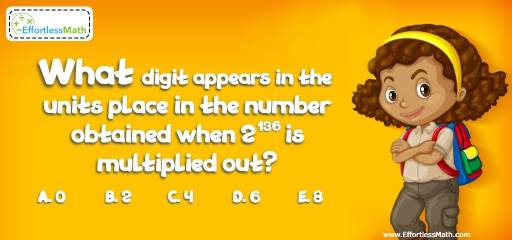# Number Properties Puzzle – Challenge 16

Enjoy exercising your brain? Looking for great math puzzles? If so, then you are in the right place.## Challenge:

What digit appears in the units place in the number obtained when $$2^{136}$$ is multiplied out?

A- 0

B- 2

C- 4

D- 6

E- 8

### The Absolute Best Book to Challenge Your Smart Student!

Try to find the pattern:
$$2^1 = 2, 2^2 = 4, 2^3 = 8, 2^4 = 16, 2^5 = 32, 2^6 = 64$$,
The pattern repeats every 4 numbers. Therefore, when $$2^{136}$$ is multiplied out, the unit digit is 6. Why?

The Absolute Best Books to Ace Algebra

### What people say about "Number Properties Puzzle – Challenge 16 - Effortless Math: We Help Students Learn to LOVE Mathematics"?

No one replied yet.

X
45% OFF

Limited time only!

Save Over 45%

SAVE $40 It was$89.99 now it is \$49.99